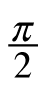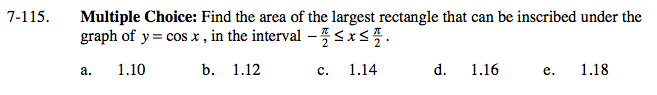### Home > CALC > Chapter 7 > Lesson 7.3.1 > Problem7-115

7-115.
1. Multiple Choice: Find the area of the largest rectangle that can be inscribed under the graph of y = cos x, in the interval −≤ x ≤. 7-115 HW eTool (Desmos). Homework Help ✎

1. 1.10

2. 1.12

3. 1.14

4. 1.16

5. 1.18Let (a, 0) be the coordinate of the lower right-hand vertex. What will be the coordinates of the other three vertices?

Write an equation for the area of this rectangle, in terms of a. Then optimize it.

Use the eTool below to help solve the problem.
Click the link at right for the full version of the eTool: Calc 7-115 HW eTool